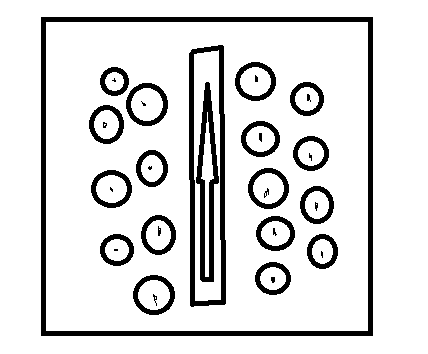Easy problem in magnetismIn the figure above, a wire (which is the oblong) is placed on a piece of paper (which is the square) in a magnetic field. If the arrow in the figure is the direction of the current through the wire and the circles indicate that the magnetic field points directly out of the paper, which way will the wire move?

×